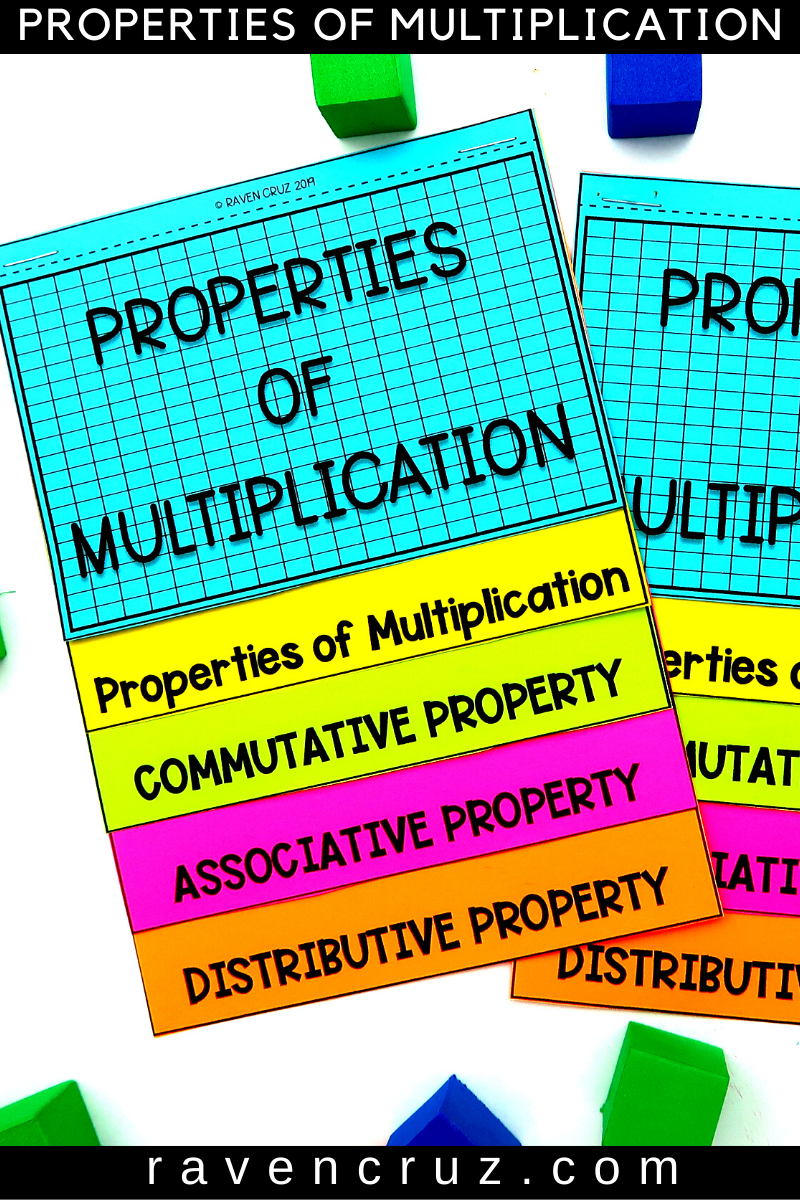November 24

# Cheap Properties of Multiplication Tools to Stop the Agony

I love flip books! I especially love quality flip books that truly help third-grade students comprehend a difficult standard. These flip books are cheap properties of multiplication tools to stop the agony in your centers, etc...

I promise, I am not tooting my own horn! These flip books guide students, step-by-step, through the commutative property, associative property, and distributive property of multiplication.## Properties of Multiplication Flip Book

As noted above, these flip books guide students through the commutative, associative, and distributive property of multiplication.

First, the definition is written in terms that students can understand.

Then, The students are shown what the definition means.

Next, a tip is given so students further conceptualize what they just read.

Last, the flip books allow students to "try it for themselves".

## Where to use the Flip Books

Many people use the flip books in interactive notebooks for students to reference when they need them. This is a great idea and, in my opinion, very beneficial.

Alternatively, I personally like the idea of putting them at math center stations and in math toolboxes. The flip books can be just as beneficial as multiplication charts.

## The Commutative Property of Multiplication

The commutative property of multiplication states that the order of the factors (numbers) does not matter the product will be the same.

For example:

5 × 7 = 7 × 5

As you can see, the numbers can move, but the answer will be the same.

## The Associative Property of Multiplication

The associative property of multiplication states that the grouping of a multiplication sentence does not matter, the product will be the same. So in other words, the parenthesis can move on each side of the number sentence, but the numbers do not change places.

For example:

3 × (5 × 7) = (3 × 5) × 7

Notice how the parenthesis are what changes the grouping in the multiplication sentence. The product will in fact be the same, and the numbers DO NOT change places.

## The Distributive Property of Multiplication

The distributive property of multiplication states that when a number is multiplied by the sum of two numbers, the first can be distributed to both of those and multiplied by each separately, then adding the two products together.

First, we "break-up" one of the factors to make it easier for 3rd-graders to multiply. If a student is given the multiplication sentence 8 × 16, it is much easier for the student to "break up" the factor 16

For example:

8 × (10 + 6)

Next, the student will distribute the 8 to the addends and write a new equation.

(8 × 10) + (8 × 6)

Last, solve the equation.

80 + 48 = 128

As you can see, "breaking-up" the factor 16 and distributing the 8 to each addend made the problem much easier to solve. A student does not necessarily have to break 16 up into 10 and 6, but it most cases it is the logical way.

If you enjoy getting FREE quality elementary math resources + tips in your email-box, sign up for my monthly freebies and newsletter below. The freebies are EXCLUSIVE to my email subscribers.

Tags

## The Back-to-School Math Freebie You Need Now

The Back-to-School Math Freebie You Need Now

## New 4th of July Math Activities that are Sure to Bring Fireworks

New 4th of July Math Activities that are Sure to Bring Fireworks